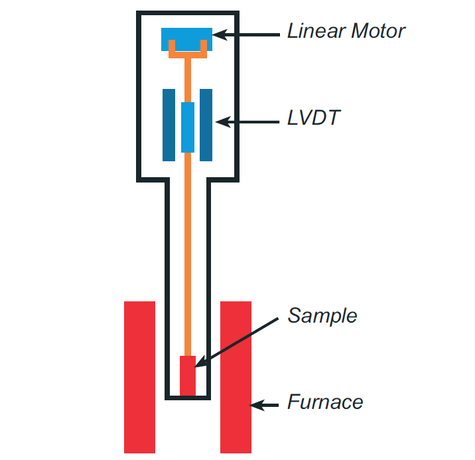## Thermomechanical Analysis

Thermomechanical Analysis (TMA) is used to characterize physical properties of materials when force is applied at specified temperatures and time periods. TMA is useful for investigating properties of viscoelastic materials, such as organic polymers. These materials exhibit both viscous and elastic properties that affect their response to mechanical stresses. For example, under conditions of ramping temperature under a fixed load, the viscoelastic material can exhibit changes in volume that correlate with changes in properties such as shrinkage, expansion, swelling and softening.

Measurements are performed by a probe that applies force to the sample. Before the test conditions are applied, the sample length is measured under a light force, which is used as the initial length (l0). As linear displacement occurs as a function of changing the applied force or temperature. The change in sample length (dl) is measured by an electrical transformer called an LVDT (linear variable displacement transducer). An example application is monitoring length change as the sample is slowly heated. In this case, the observance of a dramatic change in sample length can indicate a phase change, such as a glass transition (Tg), and the temperature at which Tg occurs can be calculated from the plot of length change versus temperature.Thermomechanical Analysis techniques involve selection of an appropriate probe type for measuring the properties of interest: melting point, softening point, glass transition, contraction (shrinkage) and expansion coefficient (CTE). Probe/technique selection is based on the category of loading best suited to the sample type and the property measurements of interest. These categories are:

Compression Force

• Measures: expansion and contraction of sample
• Measures: penetration of sample

Tension Force

• Works for films or fibers only
• Measures: expansion and contraction of sample

Either Compression or Tension Force

• Force ramp or step force to evaluate changing load on dimension change
• Isostrain: Measures the amount of force required to maintain strain at a specified constant value while material is heated

### Ideal Uses of TMA

• Measuring glass transition temperature (Tg) of polymers
• Coefficient of thermal expansion (CTE) of polymers, composites or inorganics
• Differences in CTE below and above Tg
• Differences in softening temperature before and after processing or physical aging
• Effect of post-curing on the glass transition temperature
• Differences in thermomechanical behavior of films or layered composites as a function of loading direction: “machine” and “transverse” or “in-plane” and “out-of-plane”
• Shrinkage of oriented films

### Strengths

• Small sample size
• Low force range
• Force alteration: linear and stepwise
• Programmable temperature: (1) sequential heating and cooling cycles, (2) isothermal

### Limitations

• Bulk sample size range:
• 0.5 mm up to 26 mm (z-axis, i.e. height or thickness)
• Parallel faces for expansion
• Reasonably flat for penetration
• 5 mm up to 10 mm (x -and y-dimension, i.e. width and length)
• Film/Fibers size range
• Maximum sample thickness (uniform) = 1 mm

### TMA Technical Specifications

• Temperature Operation: -150-1,000° C
• Temperature Ramp: 1-20°C/minute
• Force Range: 0.001 to 2 N (204 g)
• Operating Mode: Standard